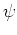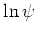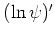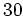Previous: 7.1.2.2 Riccati and Prüfer: Up: 7.1 Single Particle Transport Next: 7.2 The Open Von

## 7.1.3 Comparison: Schrödinger, Riccati & Prüfer Equation

For the quantum transmitting boundary method we implemented a finite difference Schrödinger solver and as equivalent alternatives a Riccati and a Prüfer solver. For the Riccati and the Prüfer solver we used a custom first order differential equation solver (the odesuite from Matlab, a sample of highly accurate ode solvers.)

The most important clue in the implementation of the Prüfer equation was the insight that one has to solve the angular equation and the equation for the amplitude in parallel. Solving for the angle first and only then for the amplitude results in jumps in the solution stemming from the minima ofwhich are not resolved, even if the error tolerance is set to the machine epsilon. However, when solving for both polar coordinates they are correctly detected and the artificial jumps in the solution disappear. In this respect the Riccati equation was better behaved, using very stringent tolerances it was always solved correctly. But it also improved when we solved forandin parallel.

All implemented methods are able to solve the Schrödinger equation with high precision. However, the Prüfer and especially the Riccati solver require quite stringent error tolerances to achieve a correct solution. This makes the solvers slow. They are at least by a factor ofslower than the Schrödinger solver and not competitive in terms of efficiency. In this last respect the Prüfer equation was superior to the Riccati equation. However, we made no attempts to implement either a special purpose Riccati or Prüfer solver, so this study may not be the last word with regard to speed comparison. But the discrete Schrödinger equation is a tridiagonal system for which there are highly optimized direct solvers. It is unlikely that one can improve on it.

The main value in the Riccati and Prüfer equation is that they allow for a check of the implementation of open boundary conditions in the Schrödinger equation. It turns out, that accuracy is excellent. The simulation results are identical for all practical purposes.

With respect to the usage of hydrodynamical methods the experiences with the Riccati equation raises some doubt that these methods can be used successfully in two dimensions. Already in one dimension it was very difficult to resolve the spikes from the minima inof the wave function.